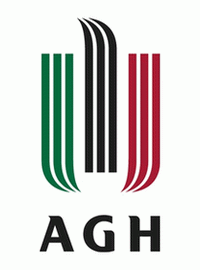Cracow, Poland# Faculty of Applied Mathematics

## Wydział Matematyki Stosowanej

Subject area: mathematics and statistics
University website: www.agh.edu.pl/en/
Mathematics has been present at the AGH University of Science and Technology since its origins. The first Rector of the University, professor Antoni Hoborski, was a mathematician. In 1969, the Departments of Mathematics and Descriptive Geometry were joined together to form the Institute of Mathematics, an interfaculty unit whose staff members taught at all university faculties. The institute became the Faculty of Applied Mathematics in 1997.
Applied Mathematics
Applied mathematics is the application of mathematical methods by different fields such as science, engineering, business, computer science, and industry. Thus, applied mathematics is a combination of mathematical science and specialized knowledge. The term "applied mathematics" also describes the professional specialty in which mathematicians work on practical problems by formulating and studying mathematical models. In the past, practical applications have motivated the development of mathematical theories, which then became the subject of study in pure mathematics where abstract concepts are studied for their own sake. The activity of applied mathematics is thus intimately connected with research in pure mathematics.
Faculty
Faculty may refer to:
Mathematics
Mathematics (from Greek μάθημα máthēma, "knowledge, study, learning") is the study of such topics as quantity, structure, space, and change. It has no generally accepted definition.
Mathematics
A marveilous newtrality have these things mathematicall and also a strange participation between things supernaturall, imortall, intellectuall, simple and indivisible, and things naturall, mortall, sensible, compounded and divisible.
John Dee, The mathematicall praeface to the Elements of geometrie of Euclid of Megara (1570) as editor of Euclid's Elements, translated by Henry Billingsley.
Mathematics
An arguing couple spiraling into negativity and teetering on the brink of divorce is actually mathematically equivalent to the beginning of a nuclear war.
Hannah Fry, The Mathematics of Love (2015), p. 104.
Applied Mathematics
Pure mathematics consists entirely of assertions to the effect that, if such and such a proposition is true of anything, then such and such another proposition is true of that thing. It is essential not to discuss whether the first proposition is really true, and not to mention what the anything is, of which it is supposed to be true. Both these points would belong to applied mathematics. We start, in pure mathematics, from certain rules of inference, by which we can infer that if one proposition is true, then so is some other proposition. These rules of inference constitute the major part of the principles of formal logic. We then take any hypothesis that seems amusing, and deduce its consequences. If our hypothesis is about anything, and not about some one or more particular things, then our deductions constitute mathematics. Thus mathematics may be defined as the subject in which we never know what we are talking about, nor whether what we are saying is true. People who have been puzzled by the beginnings of mathematics will, I hope, find comfort in this definition, and will probably agree that it is accurate.
Bertrand Russell, Recent Work on the Principles of Mathematics, published in International Monthly, Vol. 4 (1901),

## Contact:

30 Mickiewicza Av.
30-059 Krakow
Centre for International Students

Regular studies
P: +48 12 617 50 92
P: +48 12 617 46 15
F: +48 12 617 52 39
E: international.students@agh.edu.pl

Exchange programmes
P: +48 12 617 52 37
P: +48 12 617 52 38
F: +48 12 617 52 39
E: exchange@agh.edu.pl